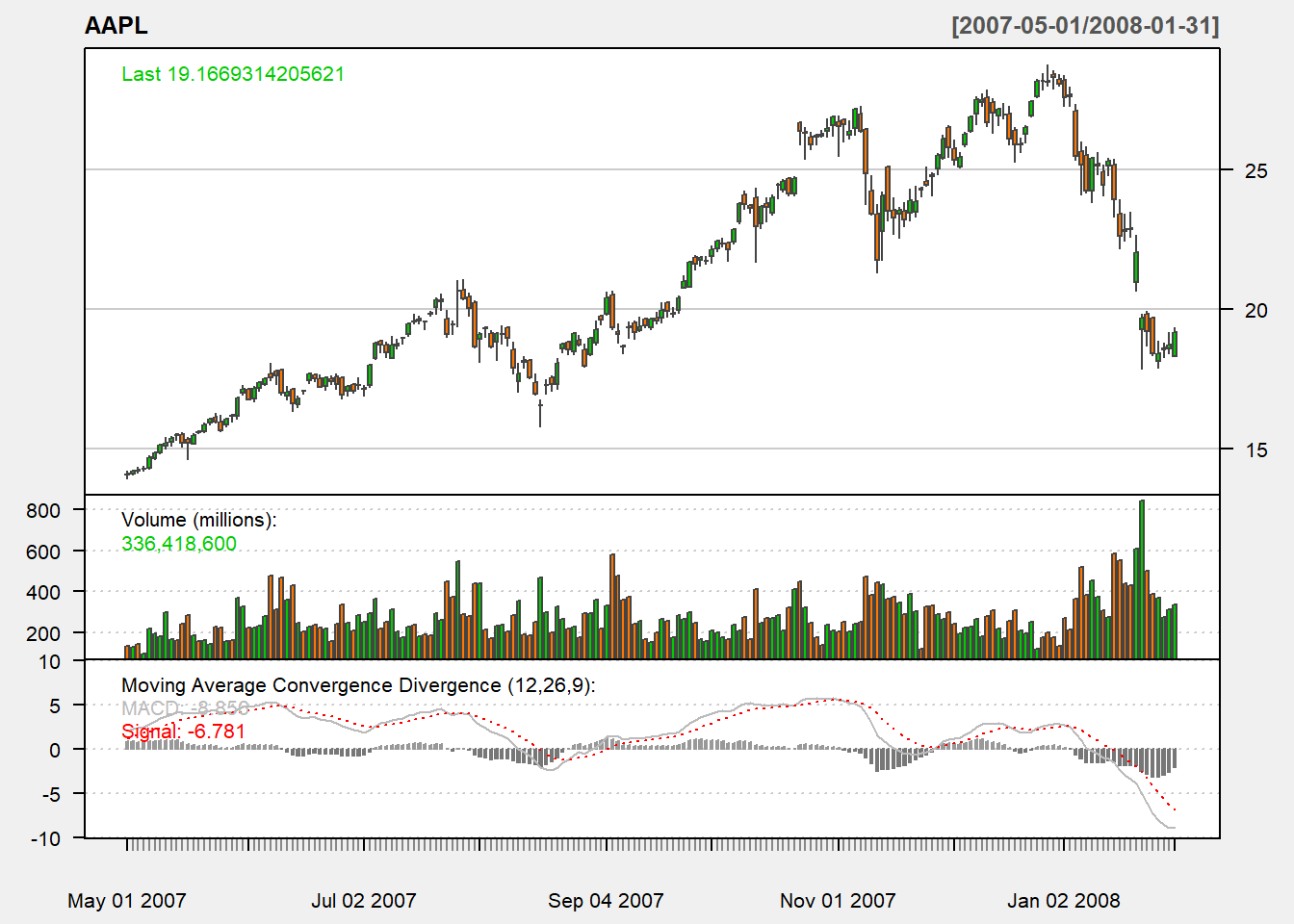## 5.9 MACD

Moving average convergence/divergence (MACD) is the difference between a short-period (fast) EMA and a long-period (slow) SMA: $MACD_t(S,L)=ema_t(P, S)-ema_t(P, L).$

For its usage, we compare it with its exponential smoothed line. This line is called the : $sig_{t}(S,L,K)=ema_t(MACD(S,L), K).$ Usually, $$K=9$$ days, $$S=12$$ days and $$L=26$$ days. Note that MACD sometimes appear as the percetnage format $MACD_t(S,L)= ema_t(P, S)-ema_t(P, L))/ema_t(P, L).$

Here is the short code for MACD:

myMACD <- function (price,S,L,K){
MACD <- EMA(price,S) - EMA(price,L)
signal <- EMA(MACD,K)
output <- cbind(MACD,signal)
colnames(output) <- c("MACD","signal")
return(output)
}

Let us apply our code:

macd <- myMACD(Cl(AAPL), 12, 26,9)
tail(macd,n=5)
##                 MACD    signal
## 2012-12-21 -2.208678 -2.072365
## 2012-12-24 -2.178151 -2.093522
## 2012-12-26 -2.211121 -2.117042
## 2012-12-27 -2.188278 -2.131289
## 2012-12-28 -2.207780 -2.146587

### 5.9.1 TTR

We use MACD function in TTR package:

macd <- MACD(Cl(AAPL), nFast=12, nSlow=26,
nSig=9, percent=FALSE)
tail(macd,n=5)
##                 macd    signal
## 2012-12-21 -2.208678 -2.072365
## 2012-12-24 -2.178151 -2.093522
## 2012-12-26 -2.211121 -2.117042
## 2012-12-27 -2.188278 -2.131289
## 2012-12-28 -2.207780 -2.146587

where nFast, nSlow, and nSig are S, L, and K in our formula. The percent is whether MACD is in percentage form or in difference form.

chartSeries(AAPL,
addMACD(fast=12,slow=26,signal=9,type="EMA")